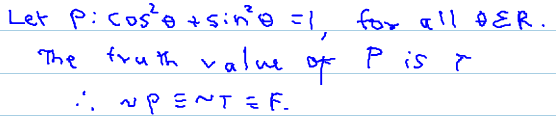9TH MAHARASHTRA: MATHS I 10TH TAMIL NADU: MATHS, SCIENCE, SSLC 10TH NEW TEXT BOOK PDF DOWNLOAD CBSE 10TH: MATHS 2019 BOARD PAPER SOLUTION: 2019 BOARD PAPER SOLUTION

LOGIC EX. NO. 1.3

Ex. No. 1.3

1. Write the negation of each of the following statements: [video]

(i) π is an irrational number.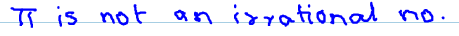(ii) 7 is greater than 5.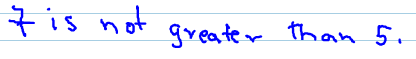(iii) Zero is not a complex number.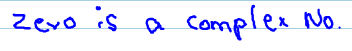(iv) It is not true that mangoes are inexpensive.2. Write the truth value of the negation of each of the following statements. [VIDEO]

(i) The sun sets in the east.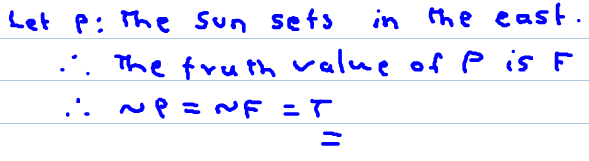(ii) Re (z) ≤ |z| , where z is a complex number.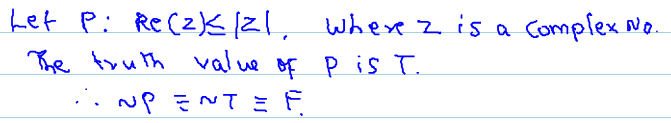(iii) Moscow is in America.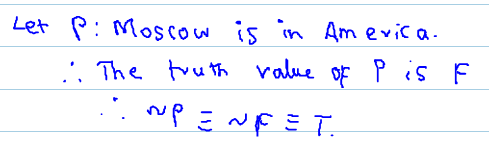(iv) cos2 θ + sin2θ = 1, for all θ Є R.